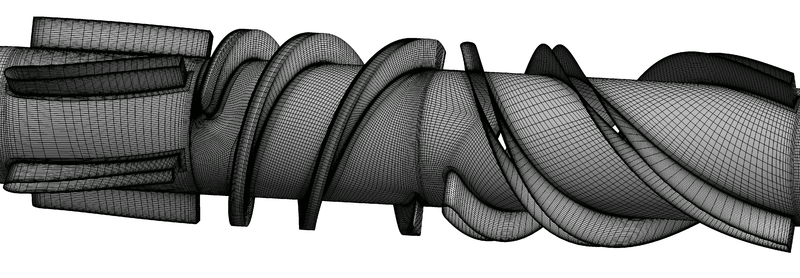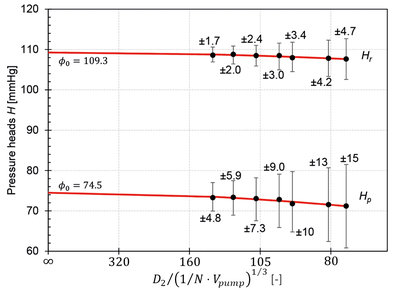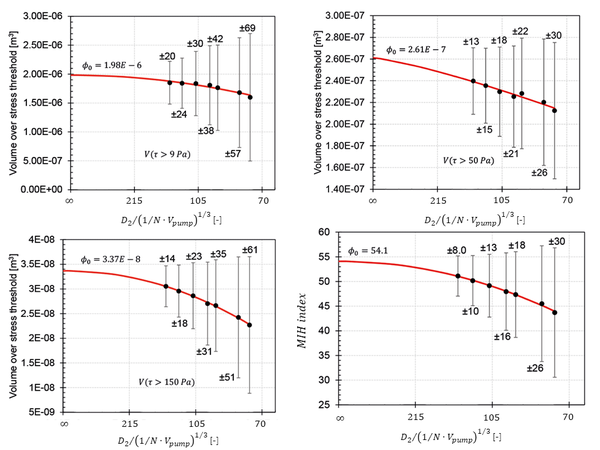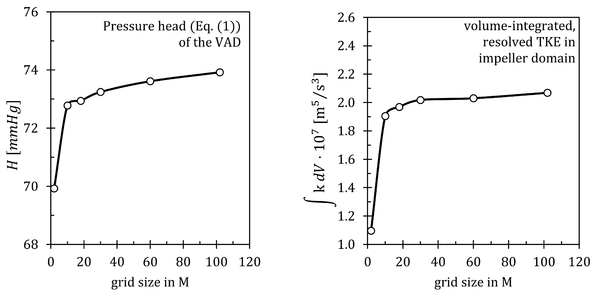# CFD Simulations

## Overview of CFD Simulations

Large-eddy simulations (LES) and unsteady Reynolds-averaged Navier-Stokes (URANS) computations were carried out using the commercial flow solver ANSYS CFX. All simulations were performed at the nominal operation (design) point and a partial operation point of the VAD. In total, six LES computations on different grid sizes were conducted for the verification of LES. The simulation on the finest grid was used as the reference case for the comparison with URANS. For the URANS cases, an extended grid convergence study was performed using seven URANS grids to analyze the influence of the spatial discretization on the main assessment parameters. The URANS computation on the finest grid was performed for the comparison with the LES results.

## Computational Domain

The whole VAD was considered in the numerical analysis. A sketch of the pump domain can be seen in Fig. 3.1. Inflow and outflow cannulas were included before and after this pump domain. The inlet and outlet of the cannulas were placed sufficiently far away (four and seven impeller diameters respectively; not shown here) from the pump in order to minimze the influences of the boundary conditions.

Block-structured grids with hexahedral-elements were created using ANSYS ICEM CFD. Since URANS and LES have different requirements for grid resolution and quality, two different, final meshes were created: a.) The final mesh for the LES computation has a size of $102M$elements. The grid was built according to literature recommendations by Fröhlich  and Menter  for wall-resolving LES methods. Attention was paid that the near-wall grid observed the upper limits of $\Delta _{x}^{+}\leq 40$for the grid size in flow direction and $\Delta _{z}^{+}\leq 15$for the grid size in spanwise direction. Furthermore, the first wall-normal node had a maximal dimensionless wall distance of $y_{1}^{+}\leq 1$and the grid expansion factor was $r_{g}=1.05$directly at the wall. This meshing strategy has been implemented throughout the whole domain. Grid angles were larger than 23$^{\circ }$and the volume change smaller than 5 with apect ratios smaller than 40 at the pump wall. The aspect ratios were further reduced with increasing wall distance, so that the values range from $1$to $5$in the core flow region. The gap was discretized with 28 and 74 elements for the height and width.Fig.3.1 Computational grid of the LES: surface grid at the blades and the hub of the rotor and guide vanes. The grid was reduced by a factor of four in each direction for better vizualisation.

The final URANS grid is coarser having a total number of $20M$elements. Mesh quality criteria were kept within the ranges of the ANSYS CFX guidelines with maximum aspect ratios around 100, volume changes smaller than 6, and a minimum angle of $20^{\circ }$. The mesh near the rotor wall has an area-averaged and maximal wall distance of $y_{1}^{+}=0.7$and $2.1$. All other pump parts have $y_{1}^{+}$-values of one or smaller. The mesh in the gap constists of 19 and 41 elements for the height and width. Additionally, the grid expansion rate was $r_{g}=1.2$. An advanced mesh convergence study of Eça and Hoekstra  was performed to check whether the mesh size is appropriate to reflect the fluid mechanical and hemodynamical parameter. For this, the final URANS mesh was coarsened to 6 coarser grids with a mesh coarsening factor between 1.06 and 1.15.

## Solution Strategy

In order to guarantee a properly working numerical scheme, several physical constraints were defined for the simulations. Blood was modeled as a Newtonian fluid with a dynamic viscosity of $\mu =3.5~mPas$and a density of $\rho =1050~kg/m^{3}$. This is a valid simplification since blood viscosity is independent of stress when shear rates are larger than $100~1/s$and this condition is fulfilled in the pump .

The impeller rotated at a constant nominal frequency of $7,900~rpm$. Transient rotor-stator coupling was used with a time step equal to $0.36^{\circ }$and $3^{\circ }$rotation of the impeller for LES and URANS, respectively. The transient rotor-stator coupling takes into account all of the transient flow characteristics by using a sliding interface, which allows for a smooth rotation between the rotary (rotor) and stationary (guide vane) part . During the calculation, the cell zones slide (that is, rotate) relative to one another along the mesh interface in discrete steps .

The resulting RMS Courant numbers were 0.6 for the Large-eddy and 3.4 for the Reynolds-averaged simulation. A total of 33 revolutions were calculated. Convergence was reached, when RMS residuals were at $10^{-5}$and all monitored values were in a statistically steady condition. Time averaging was done for at least 13 revolutions for statistical analysis of the results.

## Boundary Conditions

A constant flow rate of $Q=4.5~l/min$or $Q=2.5~l/min$were given at the outlet in the simulations. All walls were assumed to be hydraulically smooth and the no-slip condition applied. Total pressure was set to zero and a uniform velocity profile was given at the inlet. Since the Reynolds number is small at the inlet cannula (ܴ݁$Re\leq 2,300$) and no perturbations are expected upstream, no turbulent inflow condition were specified for LES.

## Application of Physical Models

For LES, the governing equations were spatially and temporarly discretized by a bounded CDS scheme and a 2nd order Euler backward scheme. The dynamic Smagorinsky sub-grid scale model was used for closure of the LES equations with a bounded Smagorinsky parameter between $C_{s}=0.0-0.3$.

For URANS a 2nd order upwind scheme and a 2nd order Euler backward scheme for spatial and temporal discretization respectively, were used. The $k$-$\omega$-SST model was applied as turbulence model. It is to be expected that streamline curvature and transitional flow effects will influence the physics within the pump. Therefore, the curvature correction model  and a $\Gamma$-$\Theta$-transition model  were applied for the $k$- $\omega$-SST model.

## Numerical Accuracy

Various numerical accuracy studies were performed with different verification methods for the URANS and LES computations (e.g., in Refs.  or ). Some of them will be presented below.

Extended Grid Convergency Study for URANS

The discretization error is a consequence of the approximation made in finite volume methods due to the transformation of the governing equations into a system of algebraic equations, which are discretely solved on a computational grid. An examination of the resulting discretization error is highly relevant and can be done for RANS methods by grid convergence studies. In this AC, the discretization error is estimated for URANS by means of an advanced grid convergence study, which determines an uncertainty interval $U_{\phi }$as an indicator for the discretization error. A brief explanation of the procedure is given below. For a detailed derivation, please see Refs.  or .

Generally, the discretization error can be estimated by:

$\epsilon _{\phi }{\widetilde {=}}\phi _{i}-\phi _{0}=\alpha h_{i}^{p},\qquad \qquad \qquad \qquad (11)$where $\phi _{i}$is an arbritary flow quantity, $\phi _{0}$is the estimate of the exact result, $\alpha$is a constant, $h_{i}=(\Delta _{x}\Delta _{y}\Delta _{z})^{1/3}$is the cell size, and $p$is the observed order of convergence.

The result of the presented method is an uncertainty interval $U_{\phi }$, which is an indicator for this discretization error $\epsilon _{\phi }$. The uncertainty is a 95% confidence interval, wherein the exact solution of the URANS equations (solution on an infinitely fine grid) is expected. The determination of $U_{\phi }$depends on several simulation and convergence properties, which are explained in detail in the study of Eça and Hoekstra .

It is possible to examine whether a computational grid is sufficiently fine or still too coarse to indicate a grid-independent and reliable solution for the analyzed parameter of interest. In total, seven meshes were used for the advanced grid convergence study. These meshes are summarised in Tab. 3.1. The term $D_{2}/(1/N\cdot V_{pump})^{1/3}$indicates the dimensionless ratio of the pump's diameter $D_{2}$to the representative grid size $h_{r}=(1/N\cdot V_{pump})^{1/3}$of a specific simulation.

Table 3.1 Names and grid size properties of the seven URANS grids.
Grid Million grid elements $N$$D_{2}/(1/N\cdot V_{pump})^{1/3}$Grid refinement parameter Time and space-averaged $y_{1}^{+}$-value over blades and maximum
UR-1 3.3 $76$1.06 1.43 (3.58)
UR-2 4.0 $81$1.15 1.25 (3.29)
UR-3 5.9 $93$1.06 1.12 (3.00)
UR-4 7.0 $99$1.11 1.00 (2.74)
UR-5 9.6 $110$1.12 0.89 (2.49)
UR-6 13.6 $123$1.12 0.79 (2.31)
UR-7 19.4 $138$- 0.68 (2.14)

Figure 3.2. shows the pressure heads via the VAD impeller and the whole pump and the uncertainties experienced. The uncertainty intervals of total pressure heads were up to 4.8% for the finest grid. From an engineering point of view, these uncertainties are in an acceptable range for the VAD design. In addition, the uncertainty for the pressure head via the whole VAD (4.8%) is higher than for the impeller alone (1.7%). The reason for this is that turbulent phenomena, e.g. detachment in the outlet guide vane, affect the pressure increase via the whole pump. Those effects may have a significant grid sensitivity and thus affect the uncertainty of the pressure head for the entire VAD. Furthermore, the relatively small uncertainties for the pressure heads suggest that the finest grid resolution is enough to guarantee a nearly grid-independent solution and no further grid refinement seems to be required based on these results.Fig. 3.2. Pressure head via the impeller (top). Pressure head via the VAD (bottom). The error bars mark the numerical uncertainties (deviations in percent).

The uncertainties for the stress-dependent MIH index (Fig. 3.3, bottom right) indicate a higher but acceptable uncertainty for the finest grid with $\pm$8%. In contrast, the coarsest grid has a two times higher uncertainty for MIH (30%) than the uncertainty for the pressure head (15%). On the other hand, the uncertainties concerning the volumes, in which certain stress thresholds are exceeded, indicate larger uncertainty intervals. These uncertainties are up to 4 times larger than those for the pump characteristics, as can be seen in the figures. Of course, the uncertainties of the different blood damage indicators will decrease with higher grid resolutions so that the absolute values will converge to a final state. But even for the finest grid, a decay of the slope of the fit in Fig. 3.3. is not obvious in the range of data obtained from the flow computations.

The URANS computations were performed with grids whose mesh sizes are comparable to current URANS blood pump simulations (e.g., in , , ). In our case, there are still discernible uncertainties for the shear-dependent quantities for these mesh sizes, although a small uncertainty is indicated for the pressure heads.Fig. 3.3. Stress-dependent variables. Upper left: Volume in the pump where stresses of 9 Pa are exceeded. Upper right: Volume in the pump where stresses of 50 Pa are exceeded. Bottom left: Volume in the pump where stresses of 150 Pa are exceeded. Bottom right: Stress-dependent MIH index.

Evaluation of the Flow Resolution of LES Using Time-Averaged Quantities

The first step was to check whether the near-wall grid is fine enough to allow no-slip conditions at the walls. This was done by determining the grid step sizes in wall units, $\Delta _{x}^{+}$, $\Delta _{z}^{+}$, $y^{+}$, using time-averaged wall shear stresses. These quantities are given above and satisfy the recommendations given in Ref.  and .

Then, the degree of flow resolution was estimated in the core flow region. One way to assess the fineness of the grid is to estimate the Kolmogorov length $\eta _{k}$by the relation:

$\eta _{k}={\Bigl (}{\frac {\nu ^{3}}{\epsilon _{turb}}}{\Bigl )}^{\frac {1}{4}},\qquad \qquad \qquad \qquad (12)$which follows from the theory of isotropic turbulence . The local ratio $\Delta /\eta _{k}$gives information about the degree of resolution of dissipative scales through the grid . $\Delta$was calculated in every grid cell by $\Delta =(\Delta _{x}\Delta _{y}\Delta _{z})^{\frac {1}{3}}$. The ratio was $\leq 5$throughout the whole flow domain, which is smaller than the ratios of $\Delta /\eta _{k}<(12-25)$defined as desirable for LES from Refs.  and .

Furthermore, frequency spectra were also used as additional tools to evaluate the physical resolution of the LES on the finest grid. These are shown, for example, in Refs.  and  for various locations in the computed flow of the rotor and outlet guide vane.Fig. 3.4. Analysis of flow variables on different grid sizes. Left: Pressure head $H$. Right: Volume-integrated, resolved turbulent kinetic energy (TKE) $k$in the impeller domain.

In addition, the variations of characteristic flow variables on different grid sizes were evaluated. This is shown in figure 3.4., where the pressure head $H$and the volume-integral of the resolved, turbulent kinetic energy (TKE) $k$was plotted against the number of grid points. The resolved TKE is defined as:

$k={\frac {1}{2}}\langle {u_{i}'u_{i}'}\rangle .\qquad \qquad \qquad \qquad (13)$As can be seen from the figure, the variation of the results on the fine grid sizes ($N>30M$grid elements) is small, indicating a convergent solution with nearly constant variables on the finest grids.

Contributed by: B. Torner — University of Rostock, Germany